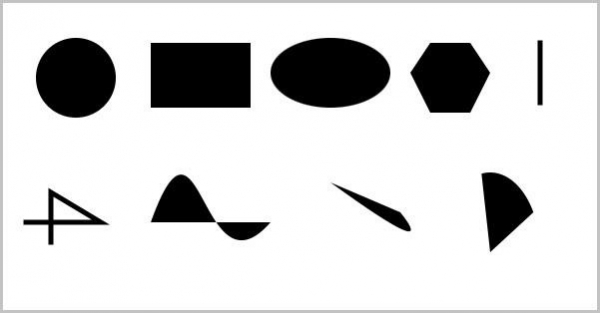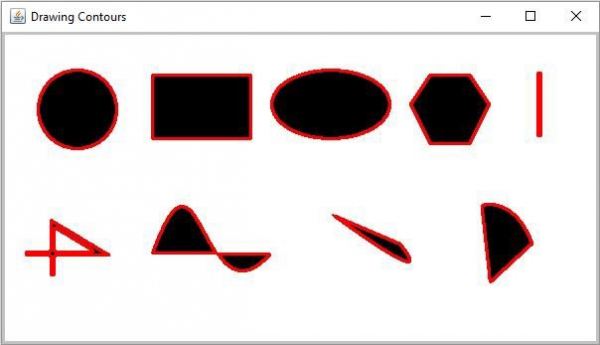# How to draw Image Contours using Java OpenCV library?

Contours is nothing but the line joining all the points along the boundary of a particular shape. Using this you can −

• Find the shape of an object.

• Calculate the area of an object.

• Detect an object.

• Recognize an object.

You can find the contours of various shapes, objects in an image using the findContours() method. In the same way you can draw

You can draw the found contours of an image using the drawContours() method this method accepts the following parameters −

• An empty Mat object to store the result image.

• A list object containing the contours found.

• An integer value specifying the contour to draw (-ve value to draw all of them).

• A Scalar object to specify the color of the contour.

• An integer value to specify the thickness of the contour.

## Example

import java.util.ArrayList;
import java.util.List;
import org.opencv.core.Core;
import org.opencv.core.Mat;
import org.opencv.core.MatOfPoint;
import org.opencv.core.Point;
import org.opencv.core.Scalar;
import org.opencv.highgui.HighGui;
import org.opencv.imgcodecs.Imgcodecs;
import org.opencv.imgproc.Imgproc;
public class DrawingContours {
public static void main(String args[]) throws Exception {
String file ="D:\Images\shapes.jpg";
//Converting the source image to binary
Mat gray = new Mat(src.rows(), src.cols(), src.type());
Imgproc.cvtColor(src, gray, Imgproc.COLOR_BGR2GRAY);
Mat binary = new Mat(src.rows(), src.cols(), src.type(), new Scalar(0));
Imgproc.threshold(gray, binary, 100, 255, Imgproc.THRESH_BINARY_INV);
//Finding Contours
List<MatOfPoint> contours = new ArrayList<>();
Mat hierarchey = new Mat();
Imgproc.findContours(binary, contours, hierarchey, Imgproc.RETR_TREE,
Imgproc.CHAIN_APPROX_SIMPLE);
//Drawing the Contours
Scalar color = new Scalar(0, 0, 255);
Imgproc.drawContours(src, contours, -1, color, 2, Imgproc.LINE_8,
hierarchey, 2, new Point() ) ;
HighGui.imshow("Drawing Contours", src);
HighGui.waitKey();
}
}

## Input Image## Output

On executing, the above program generates the following window −